• 累计撰写 270 篇文章
• 累计创建 6 个标签
• 累计收到 1 条评论

### 目 录CONTENT# 洗牌算法.md2021-08-22 / 0 评论 / 0 点赞 / 4 阅读 / 3,479 字 / 正在检测是否收录...

## 洗牌算法

@[toc]

1. 什么叫做「真的乱」？
2. 设计怎样的算法来打乱数组才能做到「真的乱」？

## 一、洗牌算法

// 得到一个在闭区间 [min, max] 内的随机整数
int randInt(int min, int max);
​
// 第一种写法
void shuffle(int[] arr) {
int n = arr.length();
/******** 区别只有这两行 ********/
for (int i = 0 ; i < n; i++) {
// 从 i 到最后随机选一个元素
int rand = randInt(i, n - 1);
/*************************/
swap(arr[i], arr[rand]);
}
}
​
// 第二种写法
for (int i = 0 ; i < n - 1; i++)
int rand = randInt(i, n - 1);
​
// 第三种写法
for (int i = n - 1 ; i >= 0; i--)
int rand = randInt(0, i);
​
// 第四种写法
for (int i = n - 1 ; i > 0; i--)
int rand = randInt(0, i);


// 假设传入这样一个 arr
int[] arr = {1,3,5,7,9};
​
void shuffle(int[] arr) {
int n = arr.length(); // 5
for (int i = 0 ; i < n; i++) {
int rand = randInt(i, n - 1);
swap(arr[i], arr[rand]);
}
}


for 循环第⼀轮迭代时， i = 0 ， rand 的取值范围是 [0, 4] ， 有 5 个可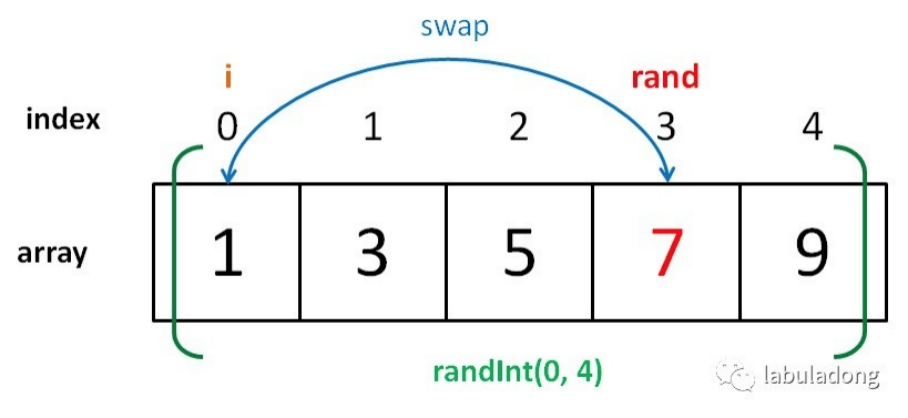for 循环第⼆轮迭代时， i = 1 ， rand 的取值范围是 [1, 4] ，有 4 个可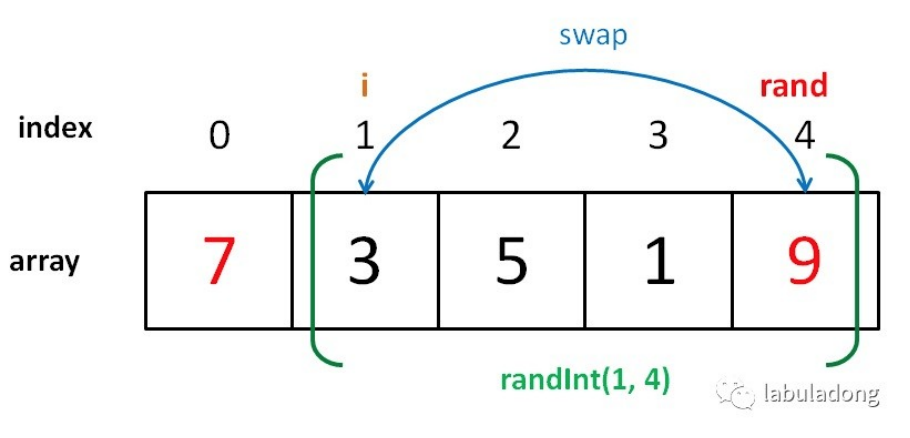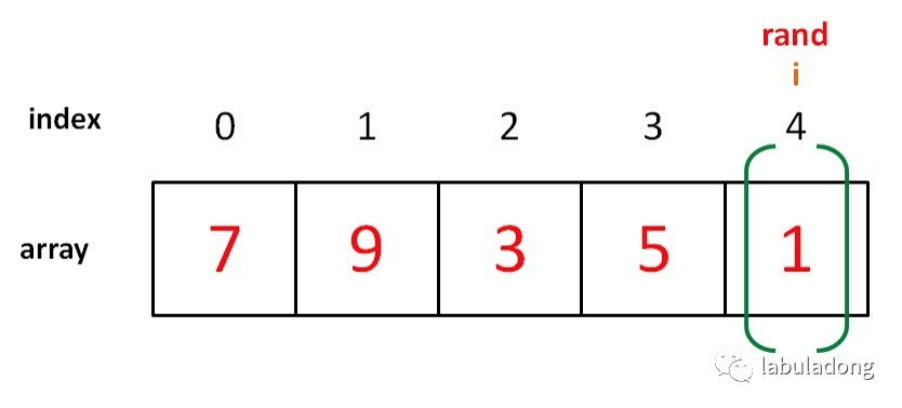分析第⼆种写法，前⾯的迭代都是⼀样的，少了⼀次迭代⽽已。所以最后⼀ 次迭代时 i = 3 ， rand 的取值范围是 [3, 4] ，有 2 个可能的取值。

// 第⼆种写法
// arr = {1,3,5,7,9}, n = 5
for (int i = 0 ; i < n - 1; i++) int rand = randInt(i, n - 1);


1 可有可⽆嘛。所以这种写法也是正确的


void shuffle(int[] arr) { int n = arr.length();
for (int i = 0 ; i < n; i++) {
// 每次都从闭区间  [0, n-1]
// 中随机选取元素进⾏交换
int rand = randInt(0, n - 1); swap(arr[i], arr[rand]);
}
}


⽐如说 arr = {1,2,3} ，正确的结果应该有 $3!= 6$ 种可能，⽽这种写法总 共有 $3^3 = 27$ 种可能结果。因为 27 不能被 6 整除，所以⼀定有某些情况 被「偏袒」了，也就是说某些情况出现的概率会⼤⼀些，所以这种打乱结果 不算「真的乱」。

## ⼆、蒙特卡罗⽅法验证正确性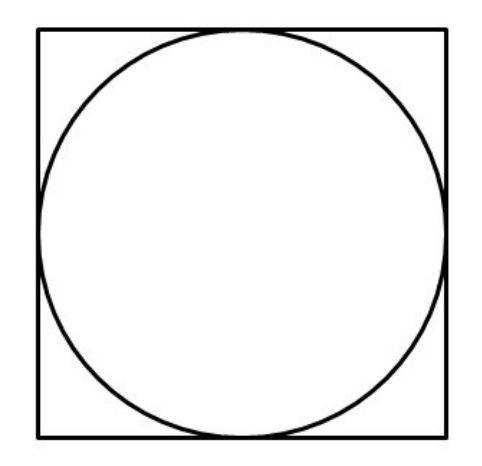（假设 arr = {1,2,3}）：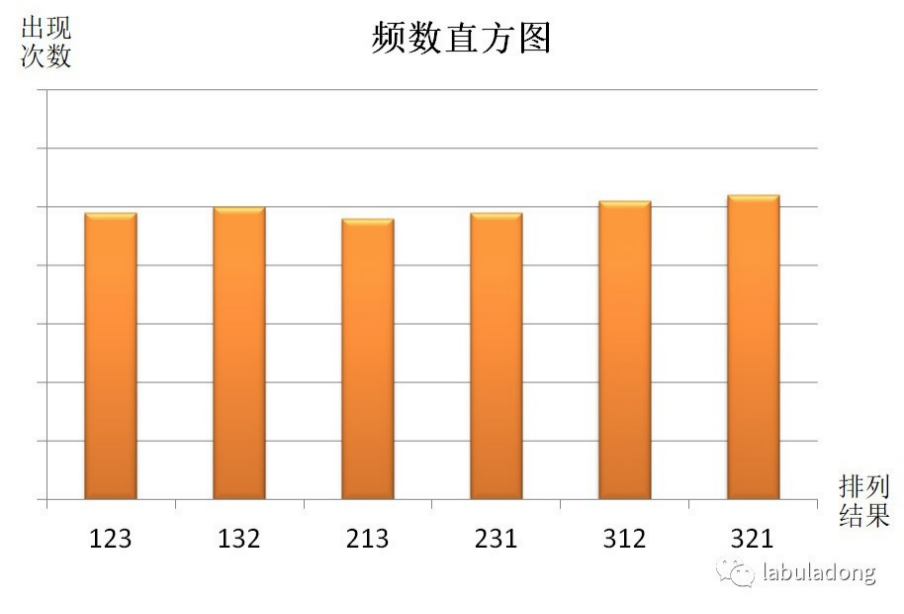void shuffle(int[] arr);

// 蒙特卡罗
int N = 1000000;
HashMap count; // 作为直⽅图
for (i = 0; i < N; i++) { int[] arr = {1,2,3}; shuffle(arr);
// 此时  arr 已被打乱
count[arr] += 1；
}
for (int feq : count.values()) print(feq / N + " "); // 频率


arr 试下就差不多了吧，因为我们只想⽐较概率验证⼀下正确性⽽已。

⾏ 100 万次打乱，记录每个索引位置出现 1 的次数，如果每个索引出现的次 数差不多，也可以说明每种打乱结果的概率是相等的。


void shuffle(int[] arr);

// 蒙特卡罗⽅法
int N = 1000000;
int[] arr = {1,0,0,0,0};
int[] count = new int[arr.length]; for (int i = 0; i < N; i++) {
shuffle(arr); // 打乱  arr
for (int j = 0; j < arr.length; j++) if (arr[j] == 1) {
count[j]++; break;
}
}
for (int feq : count)
print(feq / N + " "); // 频率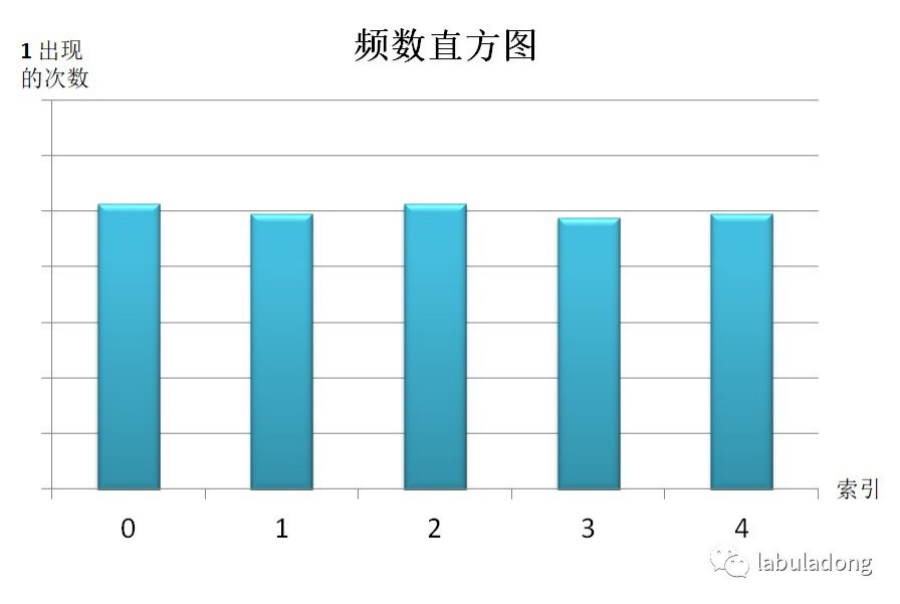⼀个在 for 循环⾥，⼀个在 for 循环之外。其实效果都是⼀样的，因为我们 的算法总要打乱 arr，所以 arr 的顺序并不重要，只要元素不变就⾏。

0